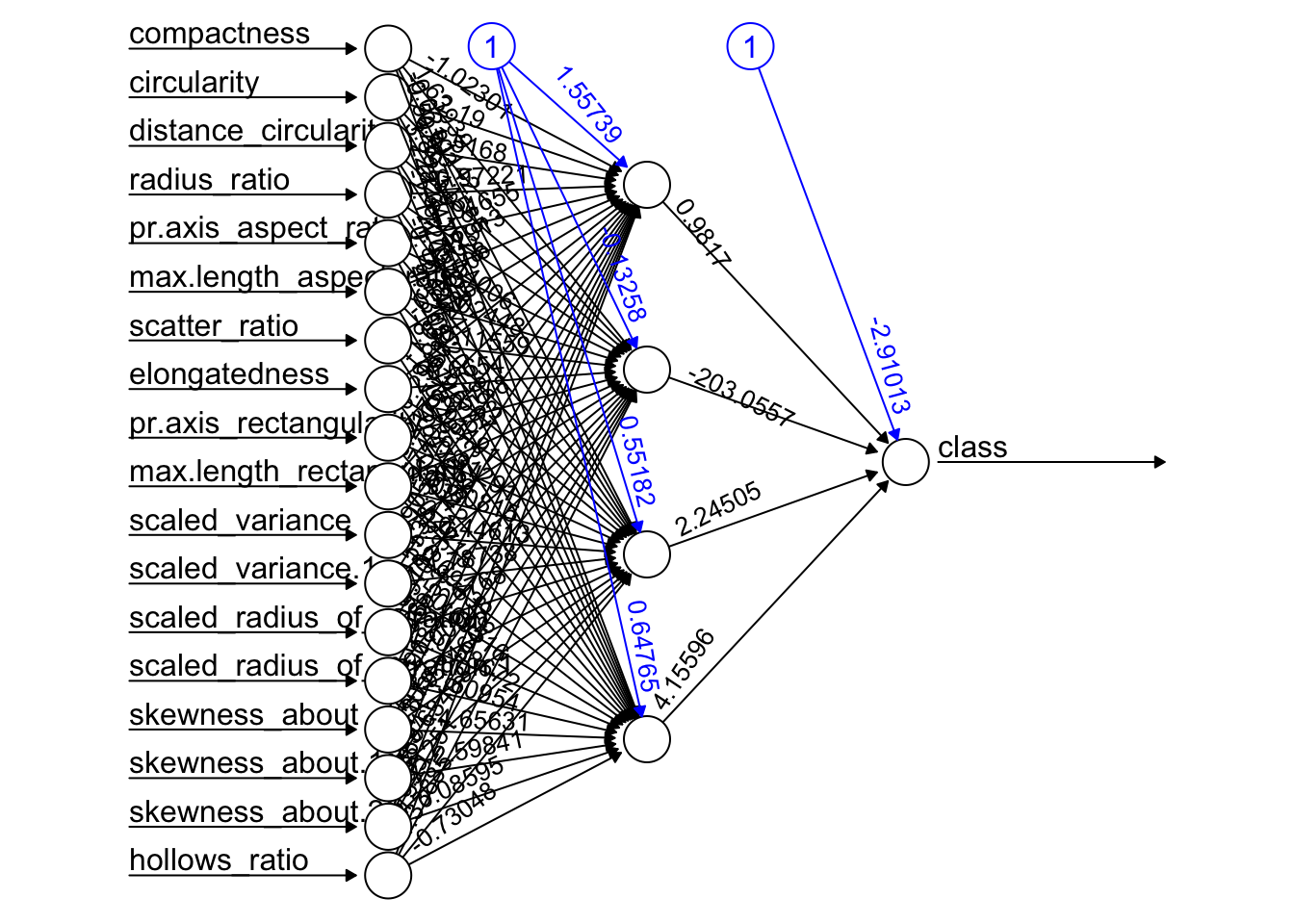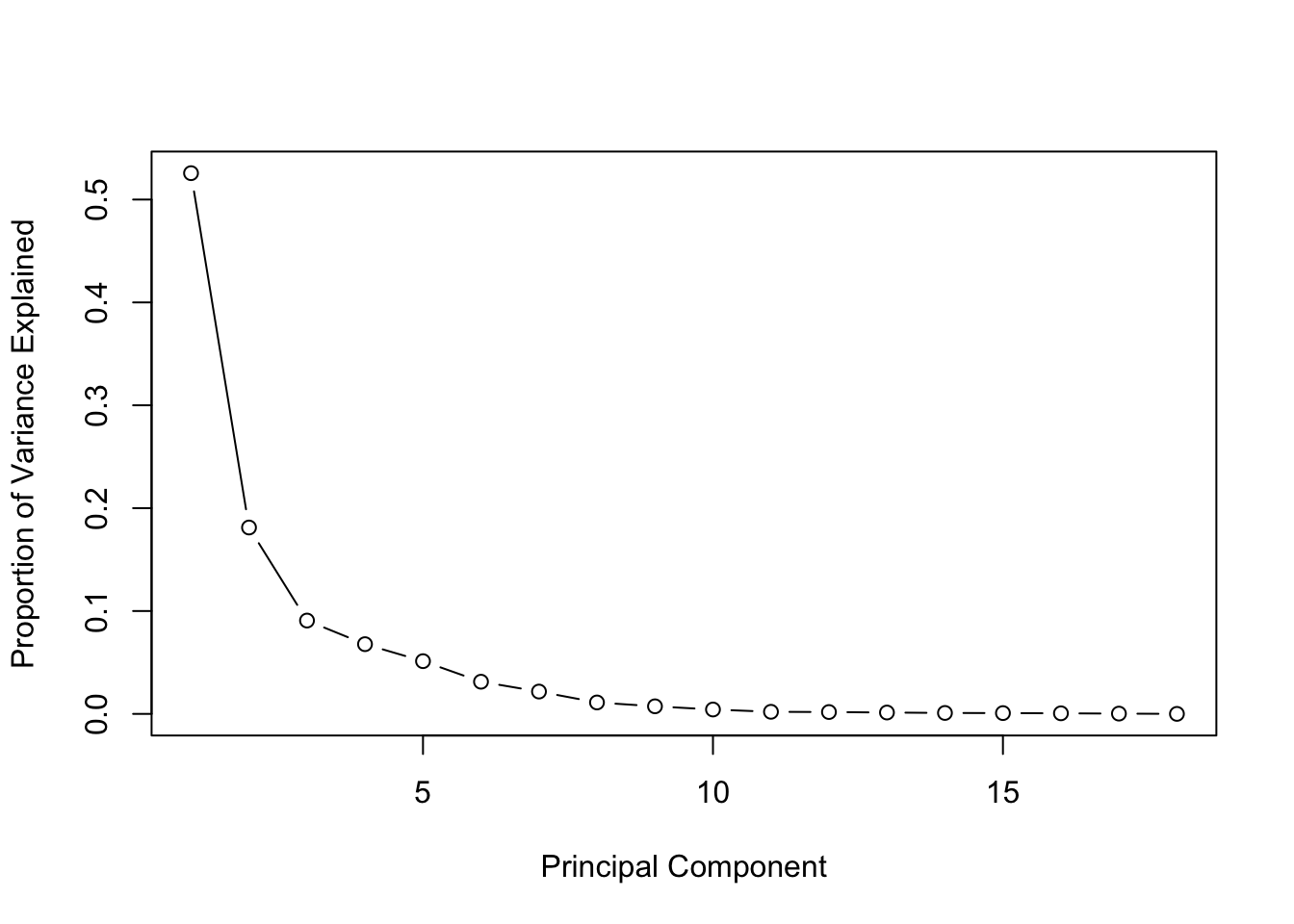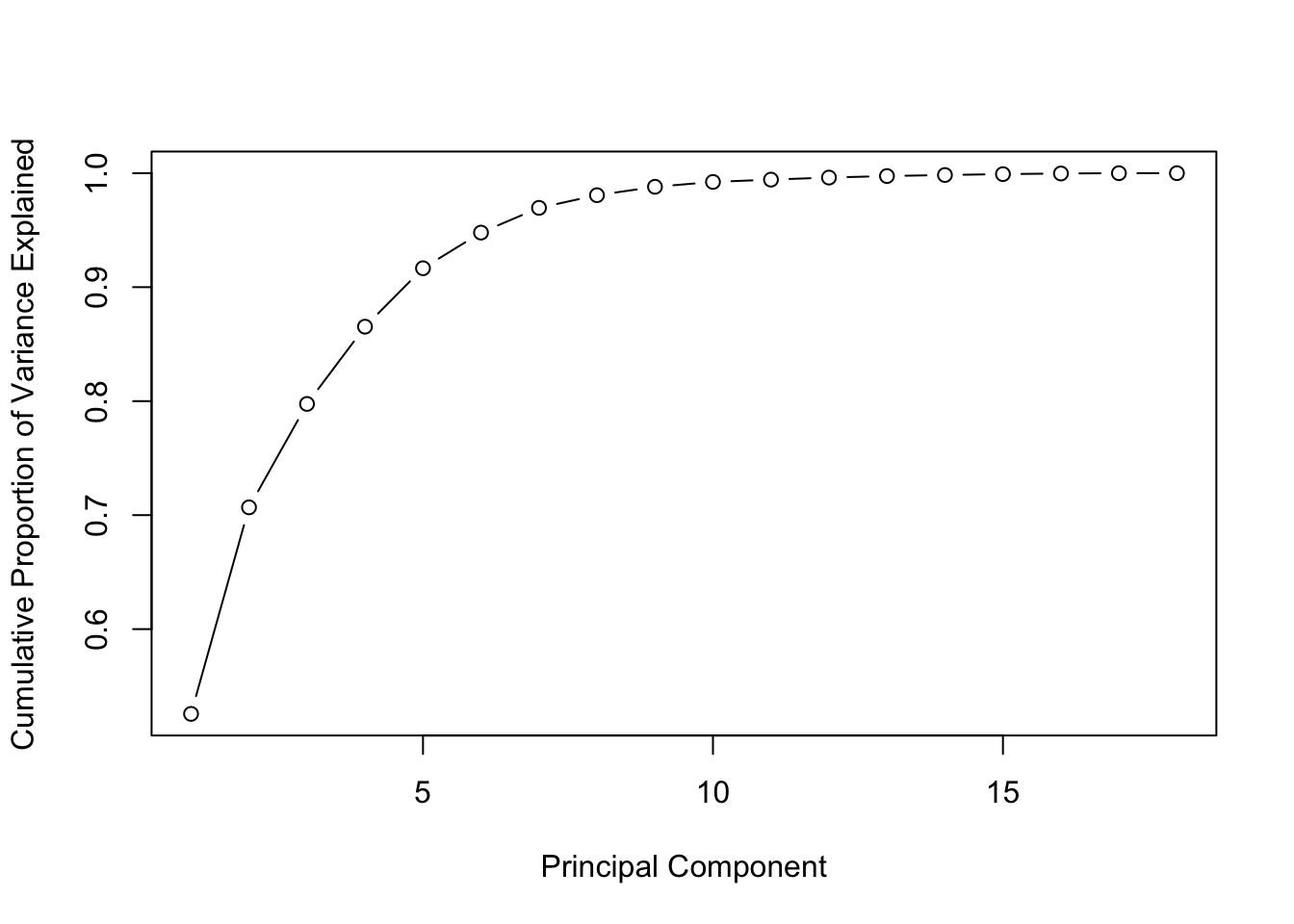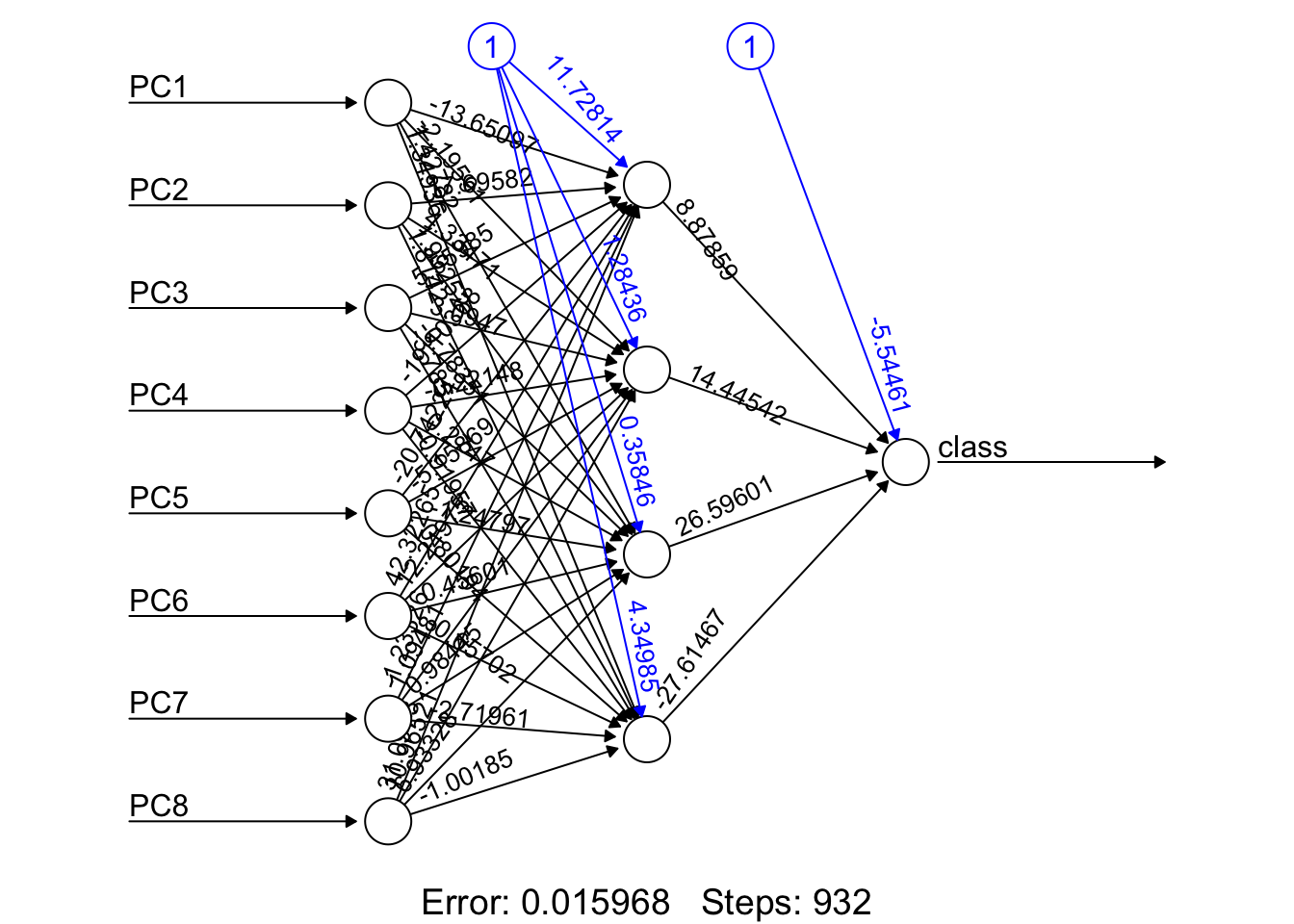Dimensionality Reduction - Does PCA really improve the outcome of classification?

PCA plus neural network with R

Posted by Meigarom Lopes on Janeiro 10, 2017

Introduction

I have come across a couple resources about dimensionality reduction techniques. This topic is definitively one of the most interesting ones, it is great to think that there are algorithms able to reduce the number of features by choosing the most important ones that still represent the entire dataset. One of the advantages pointed out by authors is that these algorithms can improve the results of classification task.

In this post, I am going to verify this statement using a Principal Component Analysis ( PCA ) to try to improve the classification performance of a neural network over a dataset. Does PCA really improve the outcome of classification? Let’s check it out.

Dimensionality reduction algorithms

Before go straight ahead to code, let’s talk about dimensionality reduction algorithms. There are two principal algorithms for dimensionality reduction: Linear Discriminant Analysis ( LDA ) and Principal Component Analysis ( PCA ). The basic difference between these two is that LDA uses information of classes to find new features in order to maximize its separability while PCA uses the variance of each feature to do the same. In this context, LDA can be consider a supervised algorithm and PCA an unsupervised algorithm.

The idea behind PCA is simply to find a low-dimension set of axes that summarize data. Why do we need to summarize data though? Let’s think about this example: We have a dataset composed by a set of properties from cars. These properties describe each car by its size, color, circularity, compactness, radius, number of seats, number of doors, size of trunk and so on. However, many of these features will measure related properties and so will be redundant. Therefore, we should remove these redundancy and describe each car with less properties. This is exactly what PCA aims to do. For example, think about the number of wheel as a feature of cars and buses, almost every example from both classes have four wheels, hence we can tell that this feature has a low variance ( from four up to six wheels or more in some of rare buses ), so this feature will make bus and cars look the same, but they are actually pretty different from each other. Now, consider the height as a feature, cars and buses have different values for it, the variance has a great range from the lowest car up to the highest bus. Clearly, the height of these vehicle is a good property to separate them. Recall that PCA does not take information of classes into account, it just look at the variance of each feature because is reasonable assumes that features that present high variance are more likely to have a good split between classes.

Often, people end up making a mistake in thinking that PCA selects some features out of the dataset and discards others. The algorithm actually constructs new set of properties based on combination of the old ones. Mathematically speaking, PCA performs a linear transformation moving the original set of features to a new space composed by principal component. These new features does not have any real meaning for us, only algebraic, therefore do not think that combining linearly features, you will find new features that you have never thought that it could exist. Many of people still believe that machine learning algorithms are magic, they put a thousands of inputs straight to the algorithm and hope to find all insights and solutions for theirs bussines. Do not be tricked. It is the job of the data scientist to locate the insights to the business through a well-conducted exploratory analysis of data using machine learning algorithm as a set of tools and not as a magic wand. Keep it in mind.

How does the principal component space looks like?

In the new feature space we are looking for some properties that strongly differ across the classes. As I showed in the previous example, some properties that present low variance are not useful, it will make the examples look the same. On the other hand, PCA looks for properties that show as much variation across classes as possible to build the principal component space. The algorithm use the concepts of variance matrix, covariance matrix, eigenvector and eigenvalues pairs to perform PCA, providing a set of eigenvectors and its respectively eigenvalues as a result. How exactly PCA performs is a material for next posts.

So, what should we do with eigenvalues and eigenvectors? It is very simple, the eigenvectors represents the new set of axes of the principal component space and the eigenvalues carry the information of quantity of variance that each eigenvector have. So, in order to reduce the dimension of the dataset we are going to choose those eigenvectors that have more variance and discard those with less variance. As we go though the example below, it will be more and more clear how exactly it works.

Let’s finally see some code

Now, we reached the fun and interesting part of this post. Let’s see if PCA really improves the result of classification task.

In order to comprove it, my strategy is to apply a neural network over a dataset and see its initial results. Afterwards, I am going to perform PCA before classification and apply the same neural network over the new dataset and last compare both results.

The dataset is originated from UCI machine learning repository called “Statlog ( Vehicle Silhouettes ) dataset”. This dataset stores some measures of four vehicles’s silhouettes with the purpose of classification. It is composed by 946 examples and 18 measures ( attributes ) all numerics values, you can check more details here in this link: https://archive.ics.uci.edu/ml/datasets/Statlog+(Vehicle+Silhouettes). The neural network will be a MultiLayer Perceptron with four hidden nodes and one output node, all with sigmoid function as activation function and PCA functions will coming from a R package.

Preparing the dataset

First of all, I am going to prepare the dataset for binary classification.

I am going to select examples only from two classes in order to make up a binary classification. The examples will come from “bus” and “saab” classes. The class “saab” will be replace by class 0 and class “bus” will be replaced by class 1. Next step consists in to separate the dataset into training and testing datasets with 60% and 40% of the total class examples, respectively.

After previous dataset preparation, let’s model a neural network using all features at once and then to apply the test dataset.

library( dplyr )

data = read.csv( "../dataset/vehicle.csv", stringsAsFactor = FALSE )

# Transform dataset
dataset = data %>%
filter( class == "bus" | class == "saab" ) %>%
transform( class = ifelse( class == "saab", 0, 1 ) )
dataset = as.data.frame( sapply( dataset, as.numeric ) )

# Spliting training and testing dataset
index = sample( 1:nrow( dataset ), nrow( dataset ) * 0.6, replace = FALSE )

trainset = dataset[ index, ]
test = dataset[ -index, ]
testset = test %>% select( -class )

# Building a neural network (NN)
library( neuralnet )
n = names( trainset )
f = as.formula( paste( "class ~", paste( n[!n %in% "class"], collapse = "+" ) ) )
nn = neuralnet( f, trainset, hidden = 4, linear.output = FALSE, threshold = 0.01 )

plot( nn, rep = "best" )# Testing the result output
nn.results = compute( nn, testset )

results = data.frame( actual = test\$class, prediction = round( nn.results\$net.result ) )

# Confusion matrix
library( caret )
t = table( results )
print( confusionMatrix( t ) )
## Confusion Matrix and Statistics
##
##       prediction
## actual  0  1
##      0 82  5
##      1 27 60
##
##                Accuracy : 0.816092
##                  95% CI : (0.7504125, 0.8706752)
##     No Information Rate : 0.6264368
##     P-Value [Acc > NIR] : 0.00000004247524
##
##                   Kappa : 0.6321839
##  Mcnemar's Test P-Value : 0.0002053757
##
##             Sensitivity : 0.7522936
##             Specificity : 0.9230769
##          Pos Pred Value : 0.9425287
##          Neg Pred Value : 0.6896552
##              Prevalence : 0.6264368
##          Detection Rate : 0.4712644
##    Detection Prevalence : 0.5000000
##       Balanced Accuracy : 0.8376853
##
##        'Positive' Class : 0
##

Results without PCA

It seems we got some results. Firstly, take a look at the confusion matrix. Basically, confusion matrix says how much examples were classified into classes. The main diagonal shows the examples that were classified correctly and secondary diagonal shows misclassification. In this first result, the classifier shows itself a bit confused because it classified correctly almost all examples from “saab” class, but it also classified most examples of “bus” class as “saab” class. Reinforcing this results, we can see that the value of accuracy is around 80%, it is a result not too bad for classification task, the classifier has essentially a probability of 80% to classify a new example correctly

Let’s see if PCA can help us

Now, let’s perform the principal component analysis over the dataset and get the eigenvalues and eigenvectors. In a practical way, you will see that PCA function from R package provides a set of eigenvalues already sorted in descending order, it means that the first component is that one with the highest variance, the second component is the eigenvector with the second highest variance and so on. The code below shows how to chose the eigenvectors looking at the eigenvalues.

# PCA
pca_trainset = trainset %>% select( -class )
pca_testset = testset
pca = prcomp( pca_trainset, scale = T )

# variance
pr_var = ( pca\$sdev )^2

# % of variance
prop_varex = pr_var / sum( pr_var )

# Plot
plot( prop_varex, xlab = "Principal Component",
ylab = "Proportion of Variance Explained", type = "b" )# Scree Plot
plot( cumsum( prop_varex ), xlab = "Principal Component",
ylab = "Cumulative Proportion of Variance Explained", type = "b" )The native R function “prcomp” from stats default packages performs PCA, it returns all eigenvalues and eigenvectors needed. The first plot shows the percentage of variance of each feature. You can see that the first component has the highest variance, something value around 50% while the 8th component is around of 0% of variance. So, it indicates that we should pick up the first eight components. The second figure show an another perspective of the variance, though the cumulative sum over all the variance, you can see that the first eight eigenvalues correspond to approximately 98% of the all variance. Indeed, it is a pretty good number, it means that there is just 2% of information being lost. The biggest beneficial is that we are moving from a space with eighteen features to another one with only eight feature losing only 2% of information. That is the power of dimensionality reduction, definitively.

Now that we know the number of the features that will compose the new space, let’s create the new dataset and then model the neural network again and check if we get new better outcomes.

# Creating a new dataset
train = data.frame( class = trainset\$class, pca\$x )
t = as.data.frame( predict( pca, newdata = pca_testset ) )

new_trainset = train[, 1:9]
new_testset =  t[, 1:8]

# Build the neural network (NN)
library( neuralnet )
n = names( new_trainset )
f = as.formula( paste( "class ~", paste( n[!n %in% "class" ], collapse = "+" ) ) )
nn = neuralnet( f, new_trainset, hidden = 4, linear.output = FALSE, threshold=0.01 )

# Plot the NN
plot( nn, rep = "best" )# Test the resulting output
nn.results = compute( nn, new_testset )

# Results
results = data.frame( actual = test\$class,
prediction = round( nn.results\$net.result ) )

# Confusion Matrix
library( caret )
t = table( results )
print( confusionMatrix( t ) )
## Confusion Matrix and Statistics
##
##       prediction
## actual  0  1
##      0 83  4
##      1  8 79
##
##                Accuracy : 0.9310345
##                  95% CI : (0.8826304, 0.9638592)
##     No Information Rate : 0.5229885
##     P-Value [Acc > NIR] : < 0.00000000000000022
##
##                   Kappa : 0.862069
##  Mcnemar's Test P-Value : 0.3864762
##
##             Sensitivity : 0.9120879
##             Specificity : 0.9518072
##          Pos Pred Value : 0.9540230
##          Neg Pred Value : 0.9080460
##              Prevalence : 0.5229885
##          Detection Rate : 0.4770115
##    Detection Prevalence : 0.5000000
##       Balanced Accuracy : 0.9319476
##
##        'Positive' Class : 0
##

Well, I guess we got better results now. Let’s to examine it carefully.

The confusion matrix shows really good results this time, the neural network is committing less misclassification in both classes, it can be seen though the values of the main diagonal and also the accuracy value is around 95%. It means that the classifier has 95% chance of correctly classifying a new unseen example. For classification problems, it is a not bad result at all.

Conclusion

Dimensionality Reduction plays a really important role in machine learning, especially when you are working with thousands of features. Principal Components Analysis are one of the top dimensionality reduction algorithm, it is not hard to understand and use it in real projects. This technique, in addition to making the work of feature manipulation easier, it still helps to improve the results of the classifier, as we saw in this post.

Finally, the answer of the initial questioning is yes, indeed Principal Component Analysis helps improve the outcome of a classifier.

What is next?

As I mentioned before there are other dimensionality reduction techniques available, such as Linear Discriminant Analysis, Factor Analysis, Isomap and its variations. The ideia is explore advantages and disadvantages of each one and check its results individually and combined as well. Would LDA combined with PCA improve the classifiers outcome? Well, let’s investigate it in the next posts.

The complete code is available on my git hub repository as well as the dataset.

Thanks for your time reading this post. I really appreciated, feedbacks are always welcome.

See you guys soon.